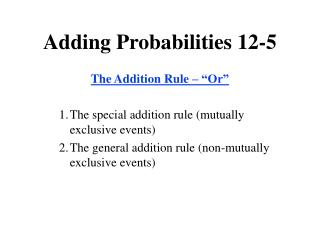# Adding Probabilities 12-5 - PowerPoint PPT PresentationDownload PresentationDownload Presentation- - - - - - - - - - - - - - - - - - - - - - - - - - - E N D - - - - - - - - - - - - - - - - - - - - - - - - - - -
##### Presentation Transcript

1. Adding Probabilities 12-5 The Addition Rule – “Or” • The special addition rule (mutually exclusive events) • The general addition rule (non-mutually exclusive events)

2. Two computer simulations of tossing a balanced coin 100 times: The Law of Large Numbers

3. Relationships Among Events (not E): The event that “E does not occur.” (A & B): The event that “both A and B occur.” (A or B): The event that “either A or B or both occur.”

4. Combinations of Events The Addition Rule – “Or” • The special addition rule (mutually exclusive events) • The general addition rule (non-mutually exclusive events)

5. Venn diagrams for (a) event (not E)(b) event (A & B) (c) event (A or B)

6. Mutually Exclusive Events Two or more events are said to be mutually exclusive if at most one of them can occur when the experiment is performed, that is, if no two of them have outcomes in common

7. Two mutually exclusive events

8. (a) Two mutually exclusive events(b) Two non-mutually exclusive events (inclusive)

9. (a) Three mutually exclusive events (b) Three non-mutually exclusive events (c) Three non-mutually exclusive events

10. Mutually Exclusive or Not? • A flipped coin: heads or tails? • A student taking a test: passing the test and getting a B? • A student taking a test: passing the test and failing it? • When rolling a six-sided die, rolling a 3 or an odd number? • When rolling a six-sided die, rolling an even or an odd number? • Choosing a card from a standard deck and it is an ace or a spade? • Choosing a card from a standard deck and it is an ace or a king?

11. The Special Addition Rule If event A and event B are mutually exclusive, then More generally, if events A, B, C, … are mutually exclusive, then That is, for mutually exclusive events, the probability that at least one of the events occurs is equal to the sum of the individual probabilities.

12. The General Addition Rule If A and B are any two events, then P(A or B) = P(A) + P(B) – P(A & B). In words, for any two events, the probability that one or the other occurs equals the sum of the individual probabilities less the probability that both occur.

13. P(A or B): Spade or Face Card

14. P(A or B): Spade or Face Card P (spade) + P (face card) – P (spade & face card) = 1/4 + 3/13 – 3/52= 22/52

15. Summary For the “Or” rule: • if the events are not mutually exclusive you have to subtract off the double count … • If the events are NOT mutually exclusive, the double count is zero. Either way, you can always use the formula: P(A or B) = P(A) + P(B) – P(A & B).